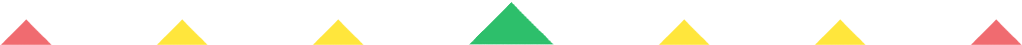Player 1
vs
Player 2
0:00
Opponent history
x
xCorrect
0
Wrong
0
Correct!
Wrong!
Help Sheet for

• 0 + 1 = 1
• 1 + 1 = 2
• 2 + 1 = 3
• 3 + 1 = 4
• 4 + 1 = 5
• 5 + 1 = 6
• 6 + 1 = 7
• 7 + 1 = 8
• 8 + 1 = 9
• 9 + 1 = 10

• 0 + 2 = 2
• 1 + 2 = 3
• 2 + 2 = 4
• 3 + 2 = 5
• 4 + 2 = 6
• 5 + 2 = 7
• 6 + 2 = 8
• 7 + 2 = 9
• 8 + 2 = 10
• 9 + 2 = 11

• 0 + 3 = 3
• 1 + 3 = 4
• 2 + 3 = 5
• 3 + 3 = 6
• 4 + 3 = 7
• 5 + 3 = 8
• 6 + 3 = 9
• 7 + 3 = 10
• 8 + 3 = 11
• 9 + 3 = 12

• 0 + 4 = 4
• 1 + 4 = 5
• 2 + 4 = 6
• 3 + 4 = 7
• 4 + 4 = 8
• 5 + 4 = 9
• 6 + 4 = 10
• 7 + 4 = 11
• 8 + 4 = 12
• 9 + 4 = 13

• 0 + 5 = 5
• 1 + 5 = 6
• 2 + 5 = 7
• 3 + 5 = 8
• 4 + 5 = 9
• 5 + 5 = 10
• 6 + 5 = 11
• 7 + 5 = 12
• 8 + 5 = 13
• 9 + 5 = 14

• 0 + 6 = 6
• 1 + 6 = 7
• 2 + 6 = 8
• 3 + 6 = 9
• 4 + 6 = 10
• 5 + 6 = 11
• 6 + 6 = 12
• 7 + 6 = 13
• 8 + 6 = 14
• 9 + 6 = 15

• 0 + 7 = 7
• 1 + 7 = 8
• 2 + 7 = 9
• 3 + 7 = 10
• 4 + 7 = 11
• 5 + 7 = 12
• 6 + 7 = 13
• 7 + 7 = 14
• 8 + 7 = 15
• 9 + 7 = 16

• 0 + 8 = 8
• 1 + 8 = 9
• 2 + 8 = 10
• 3 + 8 = 11
• 4 + 8 = 12
• 5 + 8 = 13
• 6 + 8 = 14
• 7 + 8 = 15
• 8 + 8 = 16
• 9 + 8 = 17

• 0 + 9 = 9
• 1 + 9 = 10
• 2 + 9 = 11
• 3 + 9 = 12
• 4 + 9 = 13
• 5 + 9 = 14
• 6 + 9 = 15
• 7 + 9 = 16
• 8 + 9 = 17
• 9 + 9 = 18

• 0 + 10 = 10
• 1 + 10 = 11
• 2 + 10 = 12
• 3 + 10 = 13
• 4 + 10 = 14
• 5 + 10 = 15
• 6 + 10 = 16
• 7 + 10 = 17
• 8 + 10 = 18
• 9 + 10 = 19